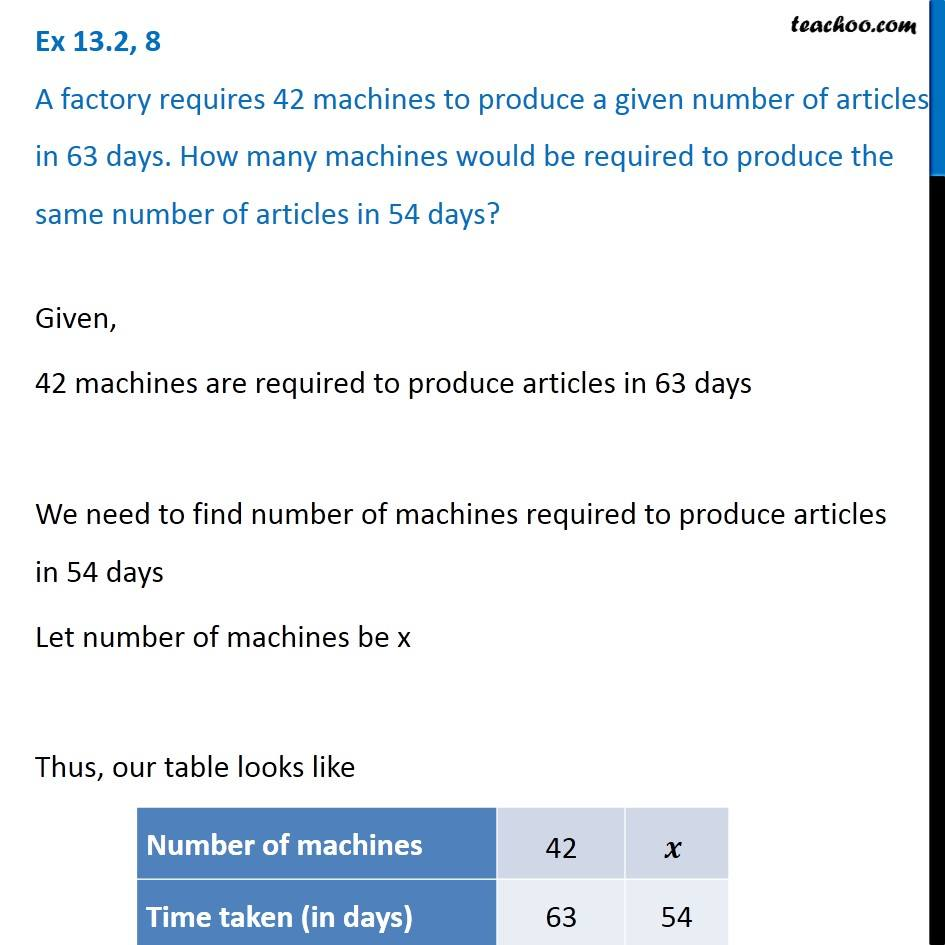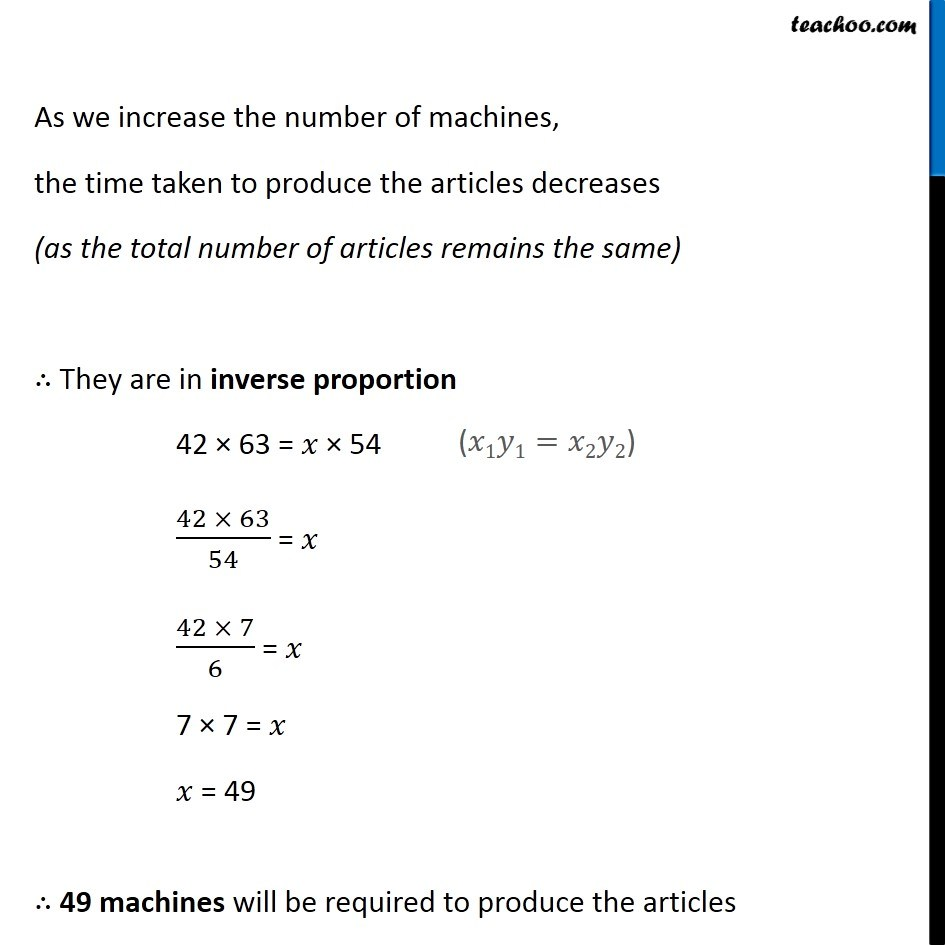1. Chapter 13 Class 8 Direct and Inverse Proportions
2. Concept wise
3. Inverse Proportion

Transcript

Ex 13.2, 8 A factory requires 42 machines to produce a given number of articles in 63 days. How many machines would be required to produce the same number of articles in 54 days? Given, 42 machines are required to produce articles in 63 days We need to find number of machines required to produce articles in 54 days Let number of machines be x Thus, our table looks like As we increase the number of machines, the time taken to produce the articles decreases (as the total number of articles remains the same) ∴ They are in inverse proportion 42 × 63 = 𝑥 × 54 (42 × 63)/54 = 𝑥 (42 × 7)/6 = 𝑥 7 × 7 = 𝑥 𝑥 = 49 ∴ 49 machines will be required to produce the articles

Inverse Proportion